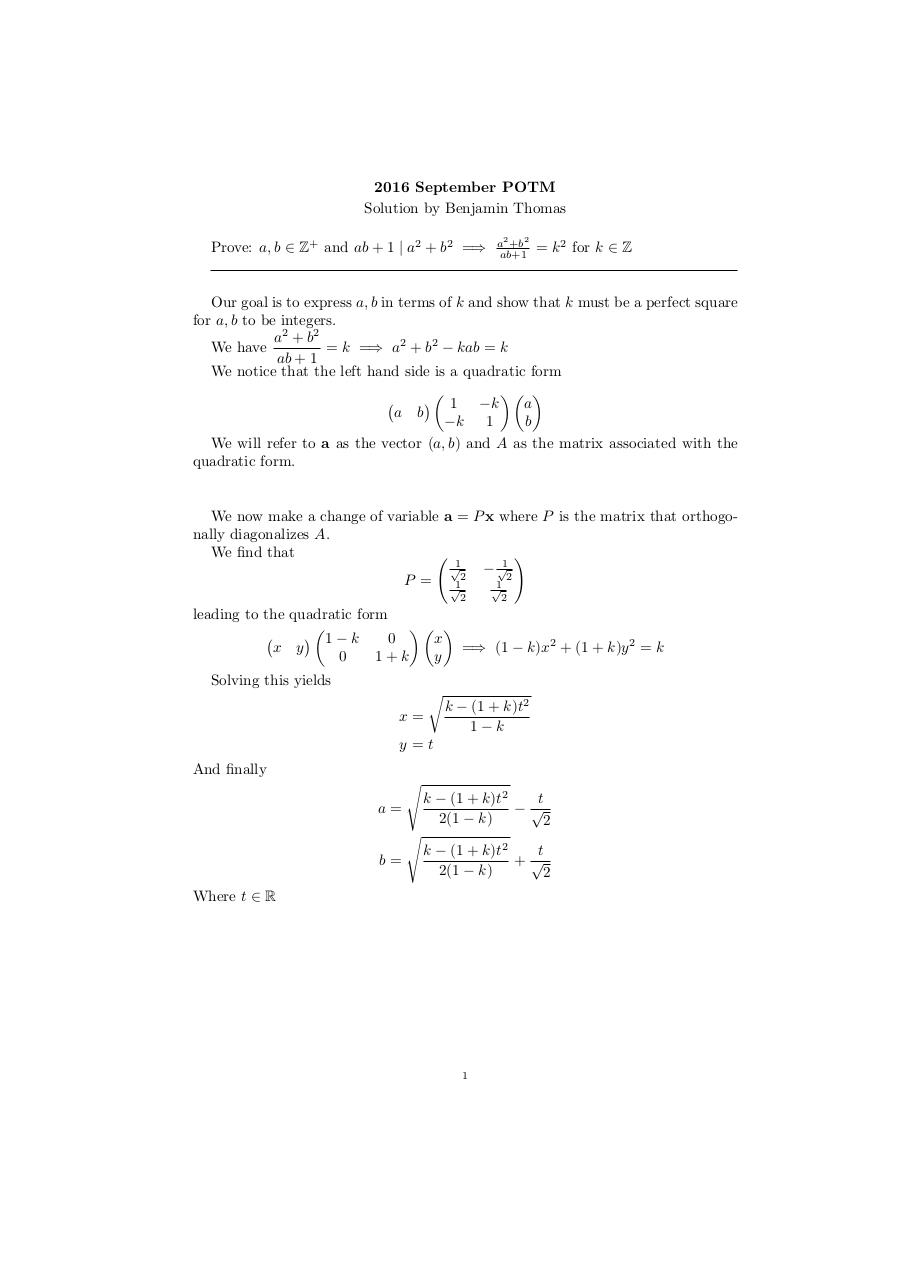# PDF Archive

Easily share your PDF documents with your contacts, on the Web and Social Networks.

## potm 2 .pdf

Original filename: potm_2.pdf

This PDF 1.5 document has been generated by TeX / pdfTeX-1.40.17, and has been sent on pdf-archive.com on 24/08/2016 at 05:36, from IP address 73.185.x.x. The current document download page has been viewed 222 times.
File size: 88 KB (1 page).
Privacy: public file### Document preview

2016 September POTM
Solution by Benjamin Thomas
Prove: a, b ∈ Z+ and ab + 1 | a2 + b2 =⇒

a2 +b2
ab+1

= k 2 for k ∈ Z

Our goal is to express a, b in terms of k and show that k must be a perfect square
for a, b to be integers.
a 2 + b2
= k =⇒ a2 + b2 − kab = k
We have
ab + 1
We notice that the left hand side is a quadratic form

1 −k
a
a b
−k 1
b
We will refer to a as the vector (a, b) and A as the matrix associated with the

We now make a change of variable a = P x where P is the matrix that orthogonally diagonalizes A.
We find that
!
√1
√1

2
P = √12
√1
2

2

1−k
0
x
x y
=⇒ (1 − k)x2 + (1 + k)y 2 = k
0
1+k
y
Solving this yields
r
x=

k − (1 + k)t2
1−k

y=t
And finally
s

k − (1 + k)t2
t
−√
2(1 − k)
2

s

t
k − (1 + k)t2
+√
2(1 − k)
2

a=

b=
Where t ∈ R

1